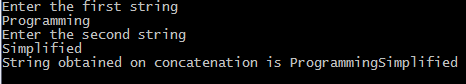## Print this Job Post## Palindrome number algorithm

1. Get the number from user.
2. Reverse it.
3. Compare it with the number entered by the user.
4. If both are same then print palindrome number
5. Else print not a palindrome number
.

## Palindrome number program c

#include <stdio.h>
#include<conio.h>
void main()
{
int n, reverse = 0, temp;
clrscr();
printf("Enter a number to check if it is a palindrome or not\n");
scanf("%d",&n);
temp = n;
while( temp != 0 )
{
reverse = reverse * 10;
reverse = reverse + temp%10;
temp = temp/10;
}
if ( n == reverse )
printf("%d is a palindrome number.\n", n);
else
printf("%d is not a palindrome number.\n", n);
getch();
}

## Tuesday, May 6, 2014

C Program to reverse a number :- This program reverse the number entered by the user and then prints the reversed number on the screen. For example if user enter 123 as input then 321 is printed as output. In our program we use modulus(%) operator to obtain the digits of a number. To invert number look at it and write it from opposite direction or the output of code is a number obtained by writing original number from right to left. To reverse or invert large numbers use long data type or long long data type if your compiler supports it, if you still have large numbers then use strings or other data structure.

## C programming code

#include <stdio.h>
#include<conio.h>
void main()
{
int n, reverse = 0;
printf("Enter a number to reverse\n");
scanf("%d",&n);
while (n != 0)
{
reverse = reverse * 10;
reverse = reverse + n%10;
n = n/10;
}
printf("Reverse of entered number is = %d\n", reverse);
getch();
}

## Swapping of two numbers in c

#include <stdio.h>
#include<conio.h>
void main()
{
int x, y, temp;
printf("Enter the value of x and y\n");
scanf("%d%d", &x, &y);
printf("Before Swapping\nx = %d\ny = %d\n",x,y);
temp = x;
x    = y;
y    = temp;
printf("After Swapping\nx = %d\ny = %d\n",x,y);
getch();
}

## Swapping of two numbers without third variable

/*You can also swap two numbers without using temp or temporary or third variable. In that case c program will be as shown :-   */

#include <stdio.h>
#include<conio.h>
void main()
{
int a, b;
printf("Enter two integers to swap\n");
scanf("%d%d", &a, &b);
a = a + b;
b = a - b;
a = a - b;
printf("a = %d\nb = %d\n",a,b);
getch();
}
To understand above logic simply choose a as 7 and b as 9 and then do what is written in program. You can choose any other combination of numbers as well. Sometimes it's a good way to understand a program.

## Swap two numbers using pointers

#include <stdio.h>
#include<conio.h>
void main()
{
int x, y, *a, *b, temp;
printf("Enter the value of x and y\n");
scanf("%d%d", &x, &y);
printf("Before Swapping\nx = %d\ny = %d\n", x, y);
a = &x;
b = &y;
temp = *b;
*b   = *a;
*a   = temp;
printf("After Swapping\nx = %d\ny = %d\n", x, y);
getch();
}

## Swapping numbers using call by reference

/*In this method we will make a function to swap numbers.*/
#include <stdio.h>
#include<conio.h>
void swap(int*, int*);
int main()
{
int x, y;
printf("Enter the value of x and y\n");
scanf("%d%d",&x,&y);
printf("Before Swapping\nx = %d\ny = %d\n", x, y);
swap(&x, &y);
printf("After Swapping\nx = %d\ny = %d\n", x, y);
getch();
}
void swap(int *a, int *b)
{
int temp;
temp = *b;
*b   = *a;
*a   = temp;
}

## C programming code to swap using bitwise XOR

#include <stdio.h>
#include<conio.h>
void main()
{
int x, y;
scanf("%d%d", &x, &y);
printf("x = %d\ny = %d\n", x, y);
x = x ^ y;
y = x ^ y;
x = x ^ y;
printf("x = %d\ny = %d\n", x, y);
getch();
}

Swapping is used in sorting algorithms that is when we wish to arrange numbers in a particular order either in ascending order or in descending.

## C programming code

#include <stdio.h>
#include<conio.h>
void main()
{
int n, sum = 0, c, value;
printf("Enter the number of integers you want to add\n");
scanf("%d", &n);
printf("Enter %d integers\n",n);
for (c = 1; c <= n; c++)
{
scanf("%d",&value);
sum = sum + value;
}
printf("Sum of entered integers = %d\n",sum);
getch();
}

## C programming code

#include <stdio.h>
#include<conio.h>
void main()
{
int first, second, add, subtract, multiply;
float divide;
printf("Enter two integers\n");
scanf("%d%d", &first, &second);
subtract = first - second;
multiply = first * second;
divide = first /second;
printf("Difference = %d\n",subtract);
printf("Multiplication = %d\n",multiply);
printf("Division = %.2f\n",divide);
getch();
}
This program concatenates strings, for example if the first string is "c " and second string is "program" then on concatenating these two strings we get the string "c program". To concatenate two strings we use strcat function of string.h, to concatenate without using library function see another code below which uses pointers.

## C programming code

#include <stdio.h>
#include <string.h>
#include<conio.h>
void  main()
{
char a, b;
printf("Enter the first string\n");
gets(a);
printf("Enter the second string\n");
gets(b);
strcat(a,b);
printf("String obtained on concatenation is %s\n",a);
getch();
}

## Output of program:String concatenation without strcat
#include <stdio.h>
#include<conio.h>
void concatenate_string(char*, char*);
void main()
{
printf("Enter source string\n");
gets(original);
printf("Enter string to concatenate\n");
printf("String after concatenation is \"%s\"\n", original);
getch();
}
{
while(*original)
original++;